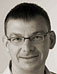# Feedback Controller Tuning

## Choosing appropriate values

In the last post, we introduced the PID controller for use in feedback loops:or, in a discrete-time software implementation:

```sum += error
output = kp * error + DT * ki * sum + kd * (error - prev) / DT
prev = error```

We also mentioned that the controller “gains” kp, ki, and kd are used to adapt the controller to the specifics of its operating environment. As an example we used a cache: its output (the metric that we want to control) is a value in the range of 0.0 to 1.0, but its input (the quantity that the controller needs to calculate) is a, possibly large, integer. We must choose appropriate values for the controller gains to adapt these numerical ranges.Here we need to make an aside: when we use the terms input and output, we are always referring to the control input and output—that is, to the quantity that we can adjust (the input), in order to control the metric that we care about (the output). These control inputs and outputs of course have nothing to do with the requests and responses coming into and leaving the cache!

The basic question we need to answer is this: by how much must we change the system’s input in order to bring about a change of a desired magnitude in the system’s output? For the caching example, this question becomes: by how much do we need to change the cache size in order to change the hit rate by (say) 0.1?

Often, the simplest (and most accurate) way to obtain the answer will be to measure it. Let’s say we operate the cache for a while (without a feedback loop!) and then we change its size, wait until the dust has settled, and compare the hit rate before and after. A first guess for the controller gain is then:

`k = (size-after - size-before)/(hitrate-after - hitrate-before)`

Let’s say that we started with a cache size of 1000 and a hit rate of 0.7, then increased the cache size to 1200 and observed a hit rate of 0.9. The gain factor then would be approximately

`k = (1200 - 1000)/(0.9 - 0.7) = 200/0.2 = 1000`

That’s not bad, as a first guess, which can be improved on manually (by adjusting the gain and observing the system’s behavior). As a general rule: larger controller gains mean faster response to changes, but less stability and an increased tendency towards instability. Consequentially, there is not one “correct” value for the controller gain—instead, it’s a typical engineering trade-off between speed and stability. Different applications will have different demands on the control strategy.

Choosing appropriate values for the controller gains (“tuning” the controller) is really important. Improperly chosen gains will lead to unsatisfactory behavior—either systems that are sluggish and respond to changes too slowly, or to systems that are not sufficiently stable and too easily perturbed. Because controller tuning is so important, various methods have been developed over the years to assist the process. None is perfect—in fact, no single one can ever be perfect, because (as we saw), optimal gain values depend not only on the system itself, but also on the way we want to use it.

One family of tuning methods is similar to the process discussed above: we apply a step change to the input of the system, and then observe the change in the system’s output. What’s different is that we observe the change in output over time. The result of such an experiment can be plotted (see Figure)—such a plot of the dynamic response to a step input is called the “process reaction curve”.This plot contains not only information about the static input/output relationship, but also information about how quickly the system will settle to its new value. The time span until the system has settled again is known as the “time constant” of the process and the controller gains must be adjusted to take it into account. Basically, systems that respond more slowly require stronger actions and people have developed various heuristic or semi-heuristic formulas that relate different properties of the curve to values for the controller gains.

Controller tuning is essential to the proper functioning of a feedback loop. Unfortunately, there is no single “correct” way to do it, and even the heuristic formulas just mentioned only provide so much guidance. It is because of this difficulties that the entire field may appear to require “art” (or even “magic”). But with a good understanding of the fundamentals, even these challenges can be overcome.

Next time, we will revisit the basic feedback idea and discuss how it is different from the more general notion of having a “self-adaptive” system. Stay tuned.

tags: